# Templates

Boost your programming skills with free Python, SQL, R, and Excel templates and create top-notch projects for your portfolio.

Save hours of browsing through the Internet and accelerate your workflow with code-ready templates for your programming projects. Personally crafted by 365 Data Science instructors, these templates bring a surprising efficiency level to your work process.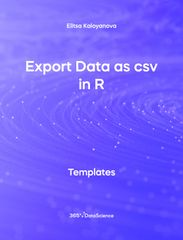Templates r

## Export Data as csv in R Template

The Export Data as csv in R template shows how to export a data set from R and save it as an csv file. The file in question contains an employee database. Some other related topics you might be interested in are Calculating Standard Deviation in R, Calculating Data Mean in R, Calculating Standard Deviation of Data in R, Exploring Data Skewness in R. You can now download the R template for free. Export Data as csv in R template is among the topics covered in detail in the 365 Data Science program.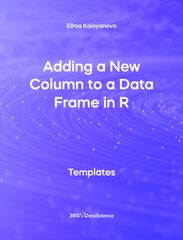Templates r

## Adding a New Column to a Data Frame in R Template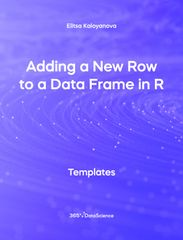Templates r

## Adding a New Row to a Data Frame in R Template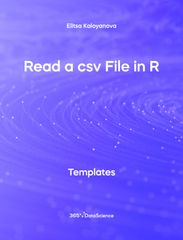Templates r

## Read a csv File in R Template

The Read a csv File in R template shows how to import a csv file into R with the help of the read csv() function. The file in question contains a real estate data set detailing information on different properties. Some other related topics you might be interested are Scatter plot with ggplot2 in R, Regression Scatter with ggplot2 in R, and Correlation between Two Variables in R. You can now download the R template for free. The Read a csv File in R template is among the topics covered in detail in the 365 Data Science program.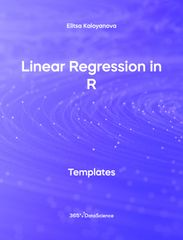Templates r

## Linear Regression in R Template

The Linear Regression in R template shows how to perform a linear regression in R using lm() on real estate property data. Some other related topics you might be interested are Scatter plot with ggplot2 in R, Regression Scatter with ggplot2 in R, and Correlation between Two Variables in R. You can now download the R template for free. Linear Regression in R template is among the topics covered in detail in the 365 Data Science program.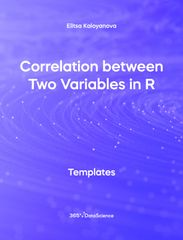Templates r

## Correlation between Two Variables in R Template

The Correlation between Two Variables in R template shows how to calculate the correlation coefficient between two variables in a dataset with the help of the corr() function. Some other related topics you might be interested in checking are Calculating Data Variance in R, Calculating Data Mean in R, Calculating Standard Deviation of Data in R, and Exploring Data Skewness in R. You can now download the R template for free. Correlation between Two Variables in R is among the topics covered in detail in the 365 Data Science program.Templates r

## While Loops in R Template

The While Loops in R shows how to create a while loop which sums up the numbers from 1 to n. A while loop operates as long as, or while its condition evaluates to TRUE. In contrast to a for loop which iterates over a sequence of number, the while loop evaluates whether a condition holds or not. Some other related topics you might be interested in exploring are For Loops in R and If Else Else If Statements in R. You can now download the R template for free. Calculating Data Variance in R is among the topics covered in detail in the 365 Data Science program.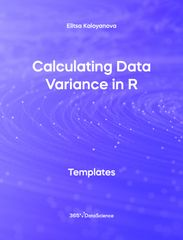Templates r

## Calculating Data Variance in R Template

The Calculating Data variance in R shows how variance measures the dispersion of a set of data points around their mean value. Some other related topics you might be interested are Calculating Standard Deviation in R, Calculating Data Mean in R, Calculating Standard Deviation of Data in R, Exploring Data Skewness in R You can now download the R template for free. Calculating Data Variance in R is among the topics covered in detail in the 365 Data Science program.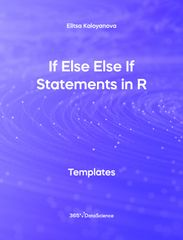Templates r

## If Else Else If Statements in R Template

The If Else Else IF Statements in R template shows whether a certain condition is met and can perform distinct actions provided the condition is met, and provided it is not. Some other related topics you might be interested in exploring are While loops in R and For Loops in R You can now download the R template for free. If Else Else If Statements in R is among the topics covered in detail in the 365 Data Science program.Templates r

## Scatter Plot with ggplot2 in R Template

The Scatter Plot with ggplot2 in R includes a color scheme from the Wes Anderson movie palette. The chart displays the relationship between the price and area of houses from a property data set. A scatter plot is one of the most widely used charts in statistics and data analytics. It shows a collection of data points across two axes, signifying two categories of the data we wish to compare. Some other related topics you might be interested are Bar chart with ggplot2 in R, Histogram with ggplot2 in R, Regression Scatter Plot with ggplot2 in R, and Line Chart with ggplot2 in R. You can now download the R template for free.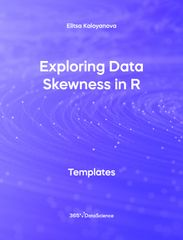Templates r

## Exploring Data Skewness in R Template

The Exploring Data Skewness in R template shows whether the observations in a data set are concentrated on one side. Here you can see examples of three different data skews implemented in R - right skewness, zero skewness and left skewness. Some other related topics you might be interested in exploring are Calculating Data Variance in R, Calculating Data Median in R, Calculating Standard Deviation of data in R. You can now download the R template for free. Calculating Data Median in R is among the topics covered in detail in the 365 Data Science program.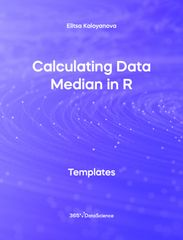Templates r

## Calculating Data Median in R Template

The following Calculating Data Median in R template shows the mean, also known as the simple average. We can find the mean of a data set by adding up all of its components and then dividing them by the number of components contained in the data set. Some other related topics you might be interested in exploring are Calculating Data Variance in R, Calculating Data Median in R, Calculating Standard Deviation of Data in R and Exploring Data Skewness in R. You can now download the R template for free. Calculating Data Median in R is among the topics covered in detail in the 365 Data Science program.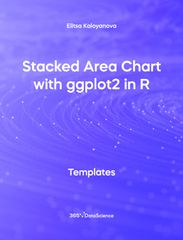Templates r

## Stacked Area Chart with ggplot2 in R Template

The following Stacked Area Chart with ggplot2 in R template shows the popularity of different engine types in automobiles across several decades. An area chart is used when we are interested in tracking and comparing the volume of a few variables over time. In a stacked area chart each category is ‘stacked’ or ‘placed’ on top of the previous and there is no overlap among categories. Some other related topics you might be interested in exploring are Line Chart with ggplot2 in R, Bar Chart with ggplot2 in R, Pie Chart with ggplot2 in R and Scatter Plot with ggpot2 in R. You can now download the R template for free. Stacked Area Chart with ggplot2 in R is among the topics covered in detail in the 365 Data Science program.Templates r

## For Loops in R Template

The following For Loops in R shows how to calculate the sum of the numbers from 1 to 10 using a for loop. For loops are one of the most commonly used tools by any programmer and they are used when we want to repeat an action in the loop for a certain number of times. Some other related topics you might be interested in exploring are While Loops in R and If Else Else If statements .Templates r

## Creating a Data Frame Object in R Template

The following Creating a Data Frame Object in R template shows you how to create a data frame in R from scratch and populate with different values. The example shows a car data frame, containing 5 columns, 3 of which are numeric and 2 of which are strings. You can use this template to create and fill out your own data frame. Some other related topics you might be interested to explore are Calculating Data Variance in R, Calculating Data Mean in R, Calculating Standard Deviation of Data in R, Exploring Data Skewness in R. You can now download the R template for free. Creating a Data Frame Object in R is among the topics covered in detail in the 365 Data Science program.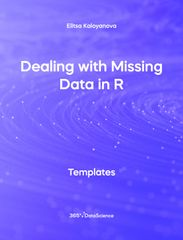Templates r

## Dealing with Missing Data in R Template

This Dealing with Missing Data in R template shows you how to check for missing values in a dataset and count them. It also provides two ways of dealing with missing values in a dataset - either by substituting the missing values with the average, or by removing the entries which have missing values in them. Some other related topics you might be interested to explore are correlation between two variables in R, export data as csv in R, calculating data median in R, calculating data mean in R, and calculating standard deviation of data in R. You can now download the R template for free. Dealing with missing data in R is among the topics covered in detail in the 365 Data Science e-learning program.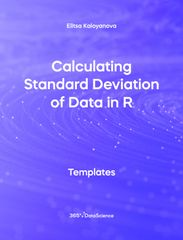Templates r

## Calculating Standard Deviation of Data in R Template

This free .r template will show you how to calculate the standard deviation of data using the R programming language. The standard deviation is obtained by taking the square root of the variance of the data. It is used in many statistical analyses, whenever we have numerical data and is a measure of data dispersion. The standard deviation shows how closely data sits around the mean – a small value shows data, which is densely populated around the mean, a large standard deviation signifies data which is more dispersed.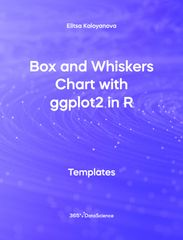Templates r

## Box and Whiskers Chart with ggplot2 in R

A box and whiskers chart graphically represents the distribution of data through their quartiles. It is often used in financial settings when analyzing the market volatility and can reveal the skewness of data or potential outliers. Тhis free .r template goes over the Titanic’s data set using the ggplot2 library in R, revealing interesting insights about e the survival rate based on age and sex. By following the outlined steps in this R template, you will learn how to convey the information professionally using the ggplot2 functionalities.